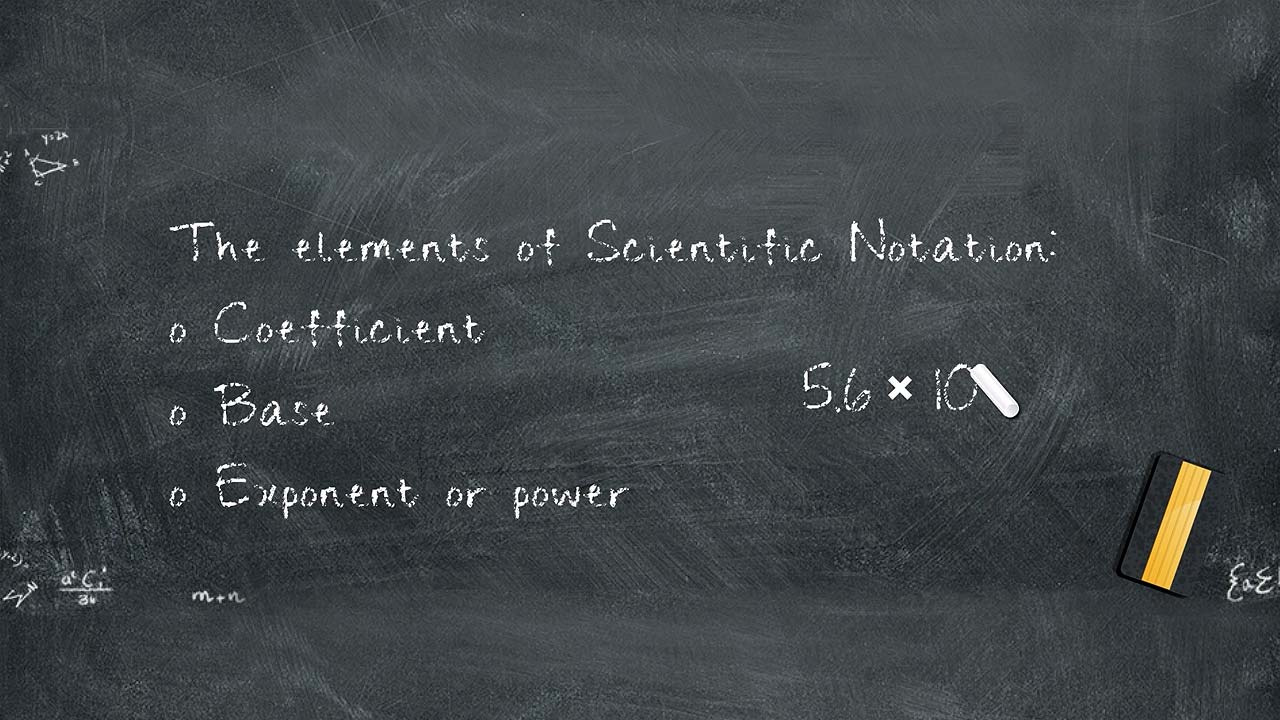# Scientific Notation

Social/Primary ImageOverview
In science, it is not unusual for us to use either very large or very small numbers in our measurements and equations. Before the advent of calculators, we used scientific or exponential notation to simplify handling these very large or very small numbers.
Objectives

Upon completion of this exercise, you will be able to:

1. Recognize why scientific notation is important
2. Describe how to represent a measurement in scientific notation
3. Convert figures between standard and scientific notation
4. Perform basic calculations using scientific notation